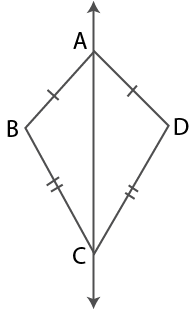# Selina Solutions Concise Maths Class 7 Chapter 17: Symmetry (Including Reflection and Rotation) Exercise 17A

Selina Solutions Concise Maths Class 7 Chapter 17 Symmetry (Including Reflection and Rotation) Exercise 17A are designed by highly experienced faculty at BYJU’S, based on ICSE syllabus. The lines of symmetry of given geometrical figures is the main concept which is covered under this exercise. The solutions contain pictorial representation in order to provide a better conceptual understanding among students. To score well in the annual exam, students can refer to Selina Solutions Concise Maths Class 7 Chapter 17 Symmetry (Including Reflection and Rotation) Exercise 17A PDF, from the links which are available below.

## Selina Solutions Concise Maths Class 7 Chapter 17: Symmetry (Including Reflection and Rotation) Exercise 17A Download PDF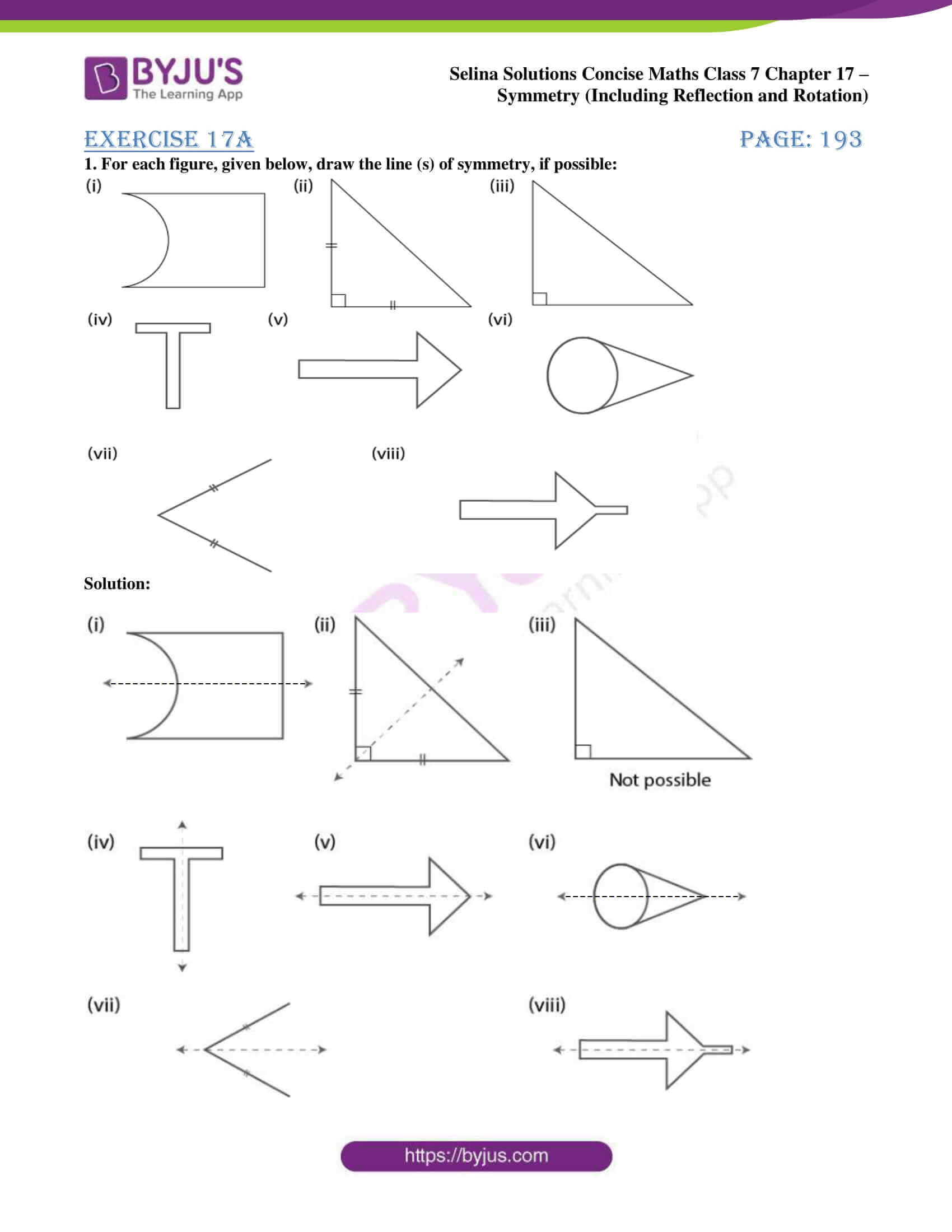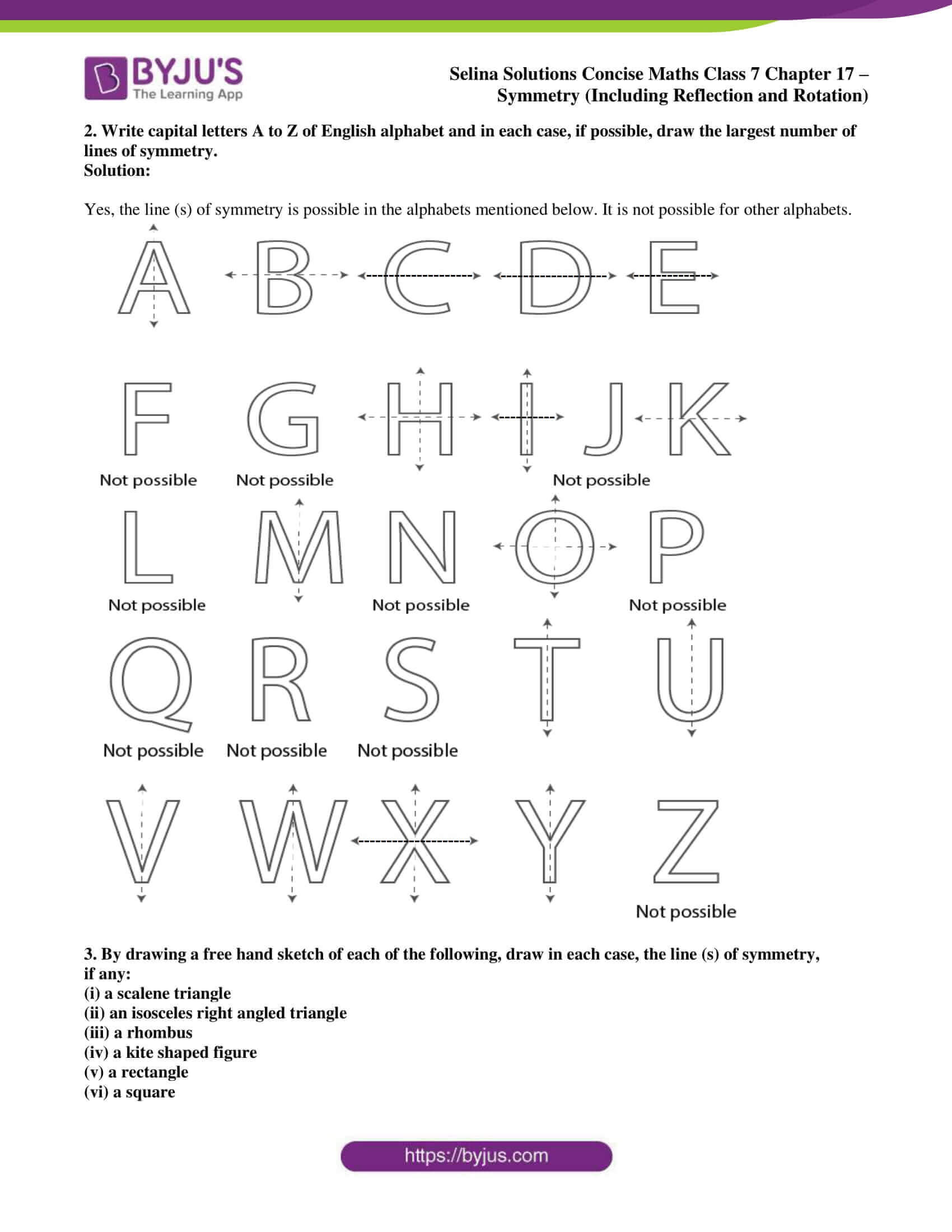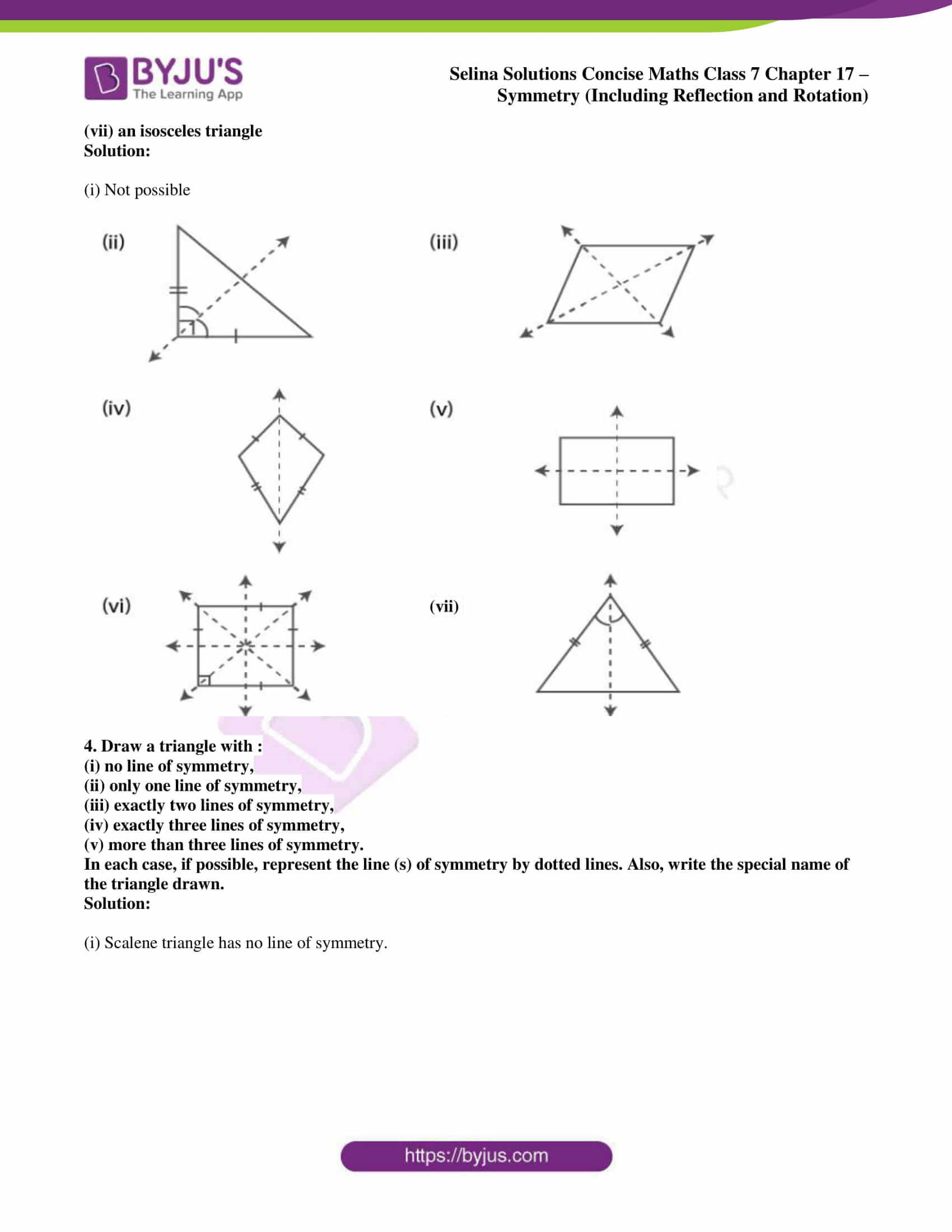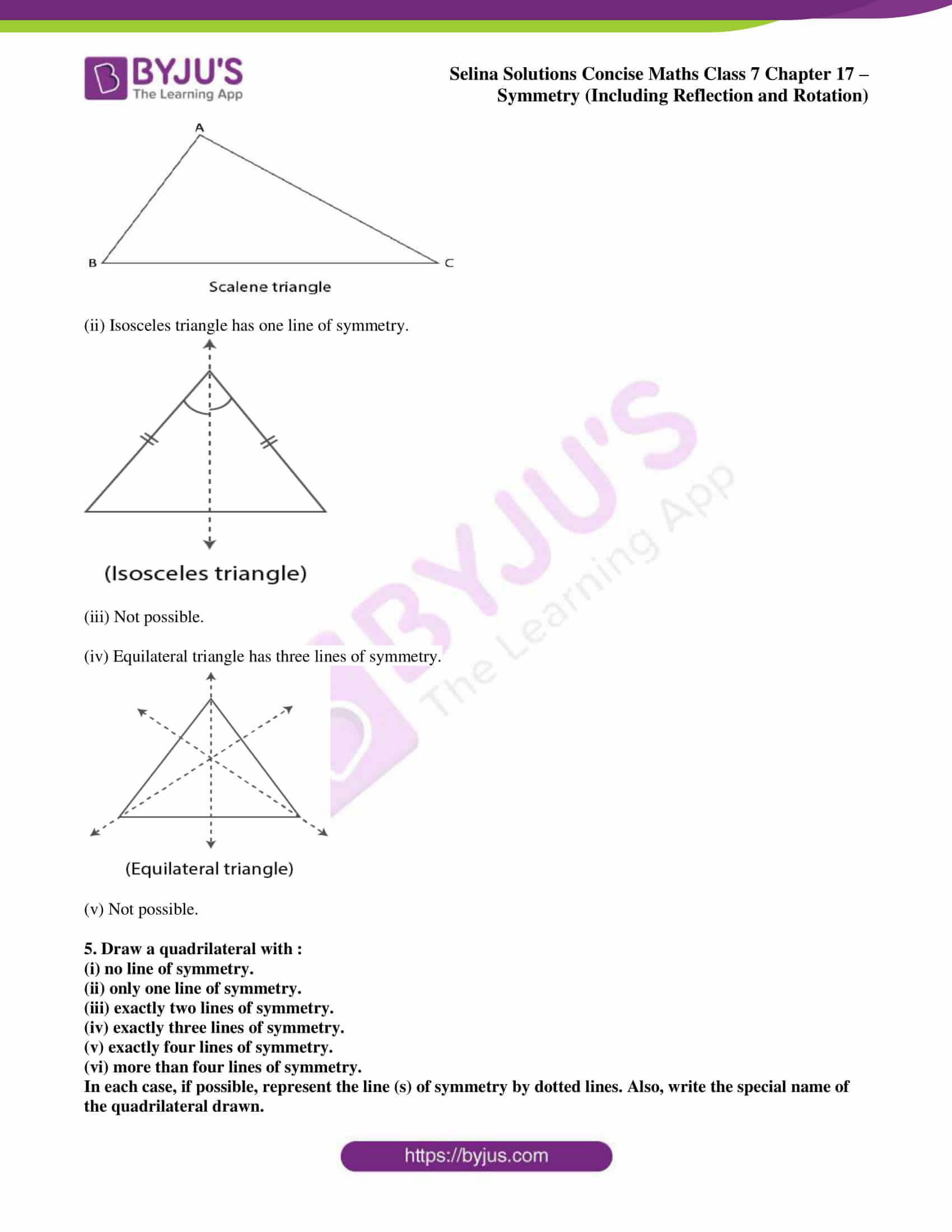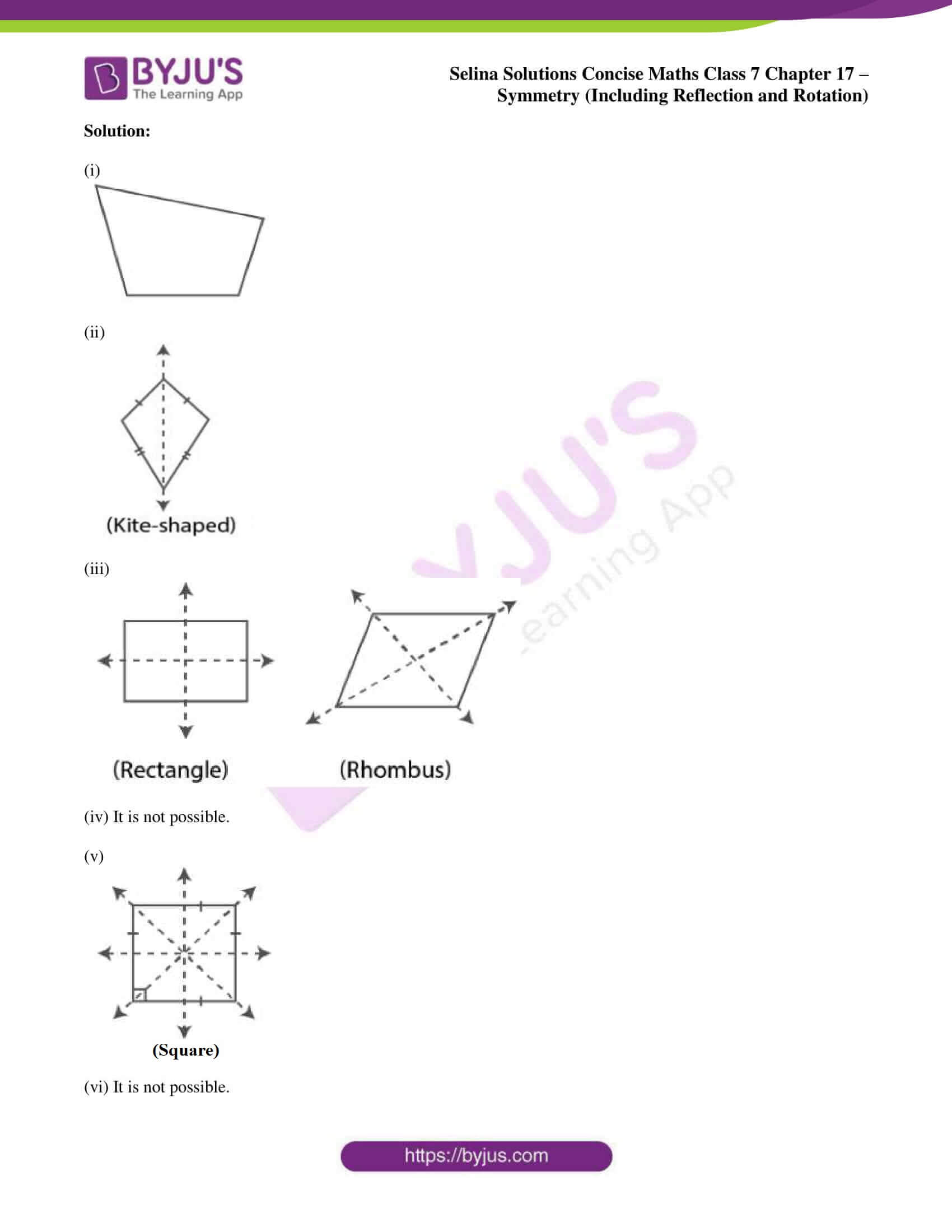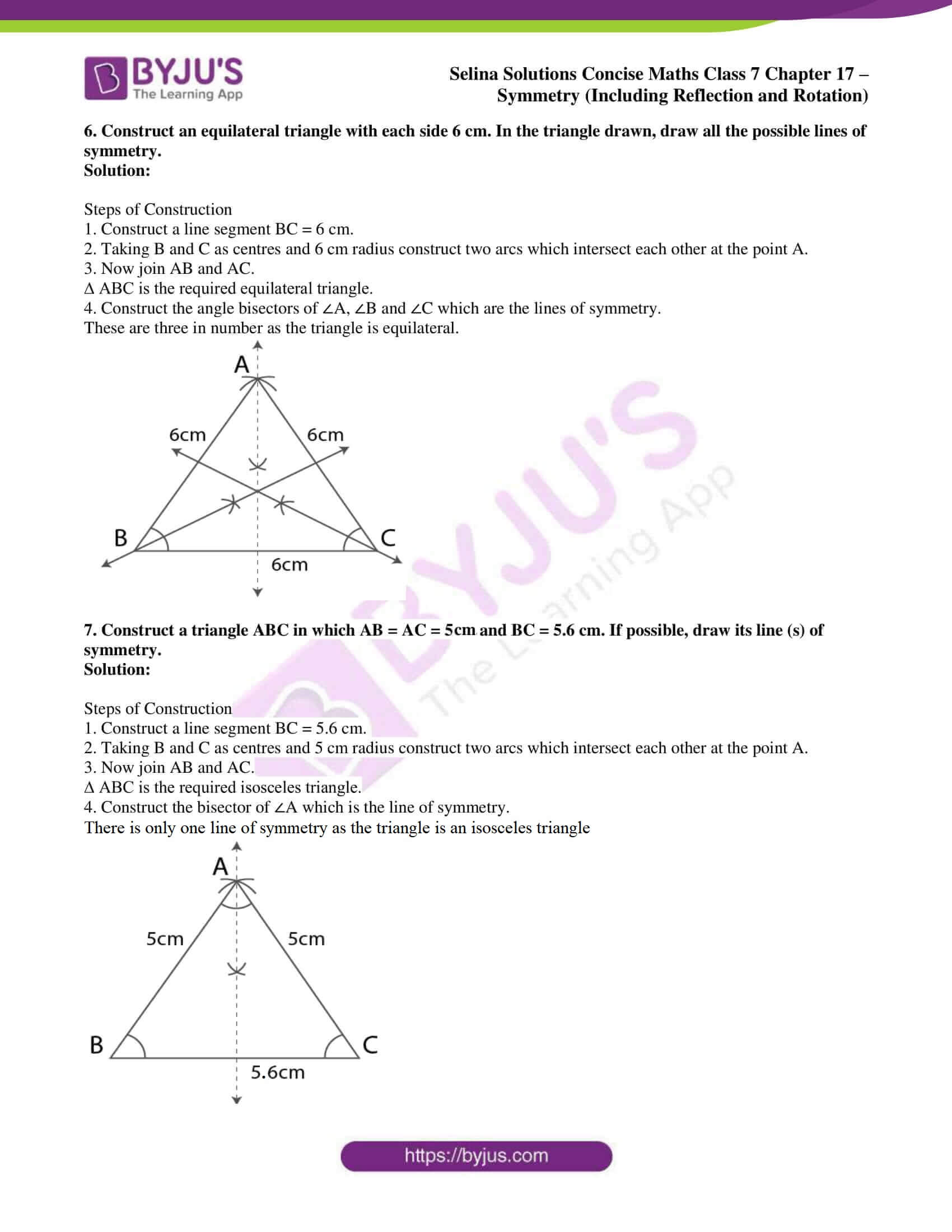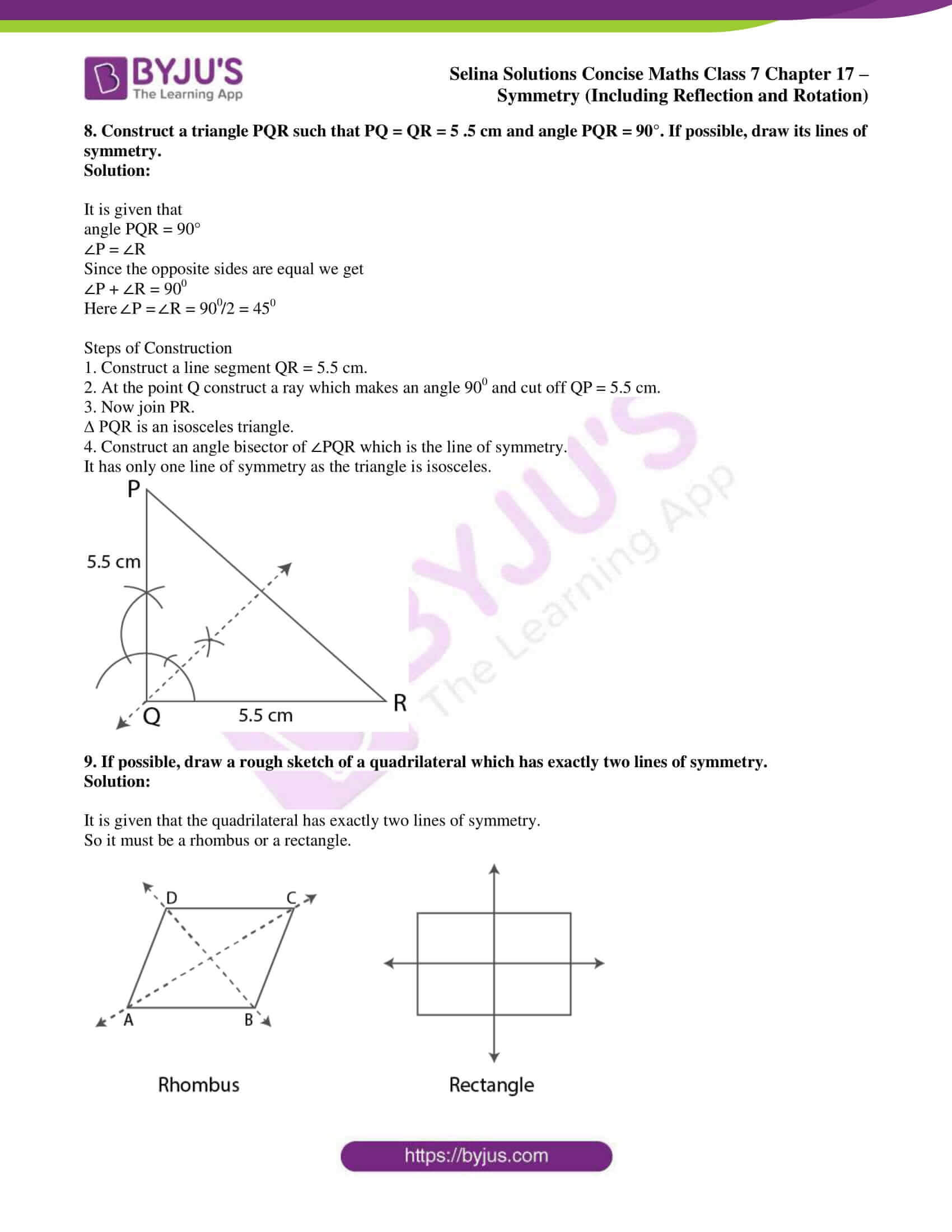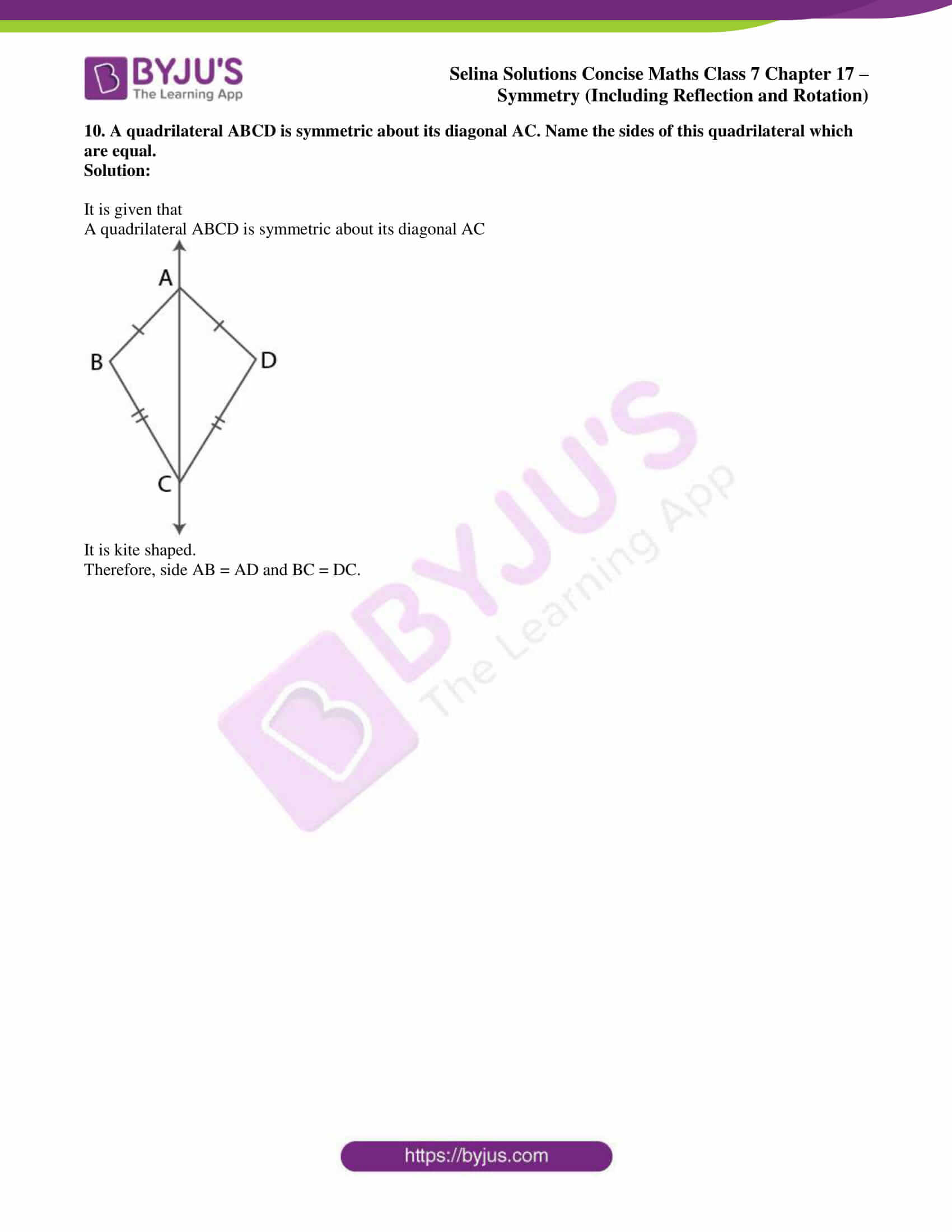### Access other exercises of Selina Solutions Concise Maths Class 7 Chapter 17: Symmetry (Including Reflection and Rotation)

Exercise 17B Solutions

Exercise 17C Solutions

### Access Selina Solutions Concise Maths Class 7 Chapter 17: Symmetry (Including Reflection and Rotation) Exercise 17A

#### Exercise 17A page: 193

1. For each figure, given below, draw the line (s) of symmetry, if possible: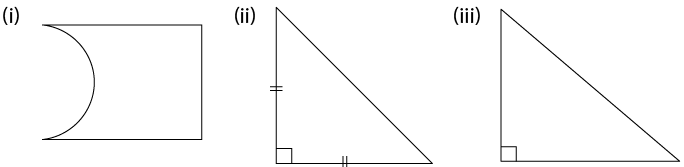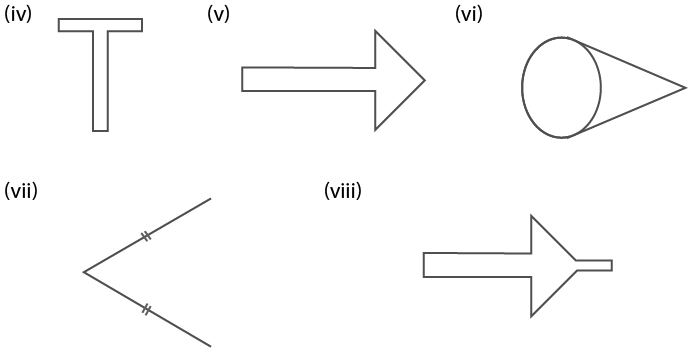Solution: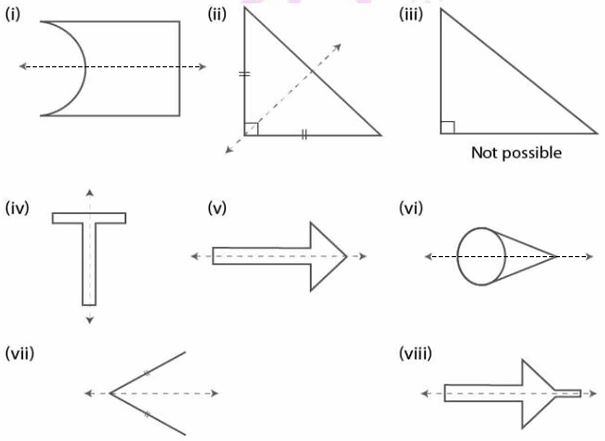2. Write capital letters A to Z of English alphabet and in each case, if possible, draw the largest number of lines of symmetry.

Solution:

Yes, the line (s) of symmetry is possible in the alphabets mentioned below. It is not possible for other alphabets.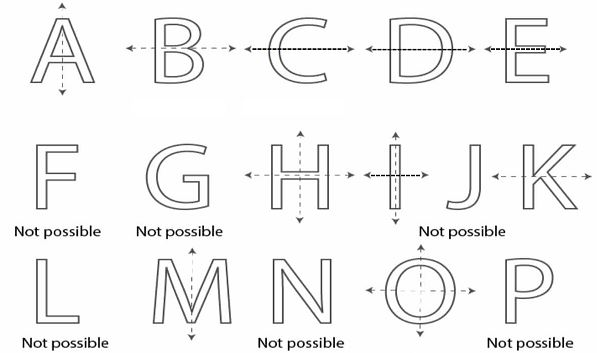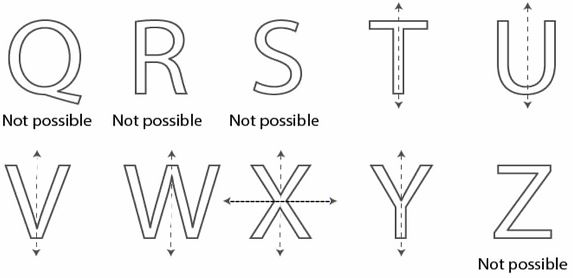3. By drawing a free hand sketch of each of the following, draw in each case, the line (s) of symmetry,

if any:

(i) a scalene triangle

(ii) an isosceles right angled triangle

(iii) a rhombus

(iv) a kite shaped figure

(v) a rectangle

(vi) a square

(vii) an isosceles triangle

Solution:

(i) Not possible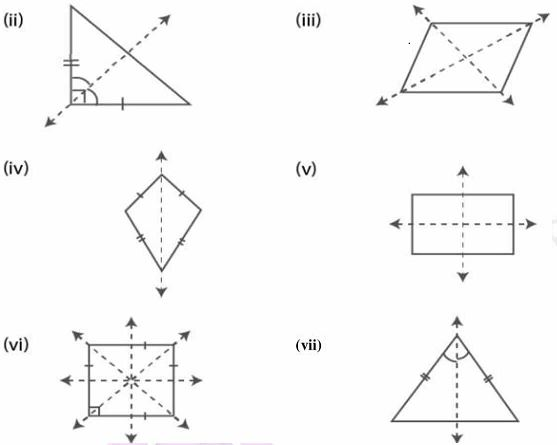4. Draw a triangle with :

(i) no line of symmetry,

(ii) only one line of symmetry,

(iii) exactly two lines of symmetry,

(iv) exactly three lines of symmetry,

(v) more than three lines of symmetry.

In each case, if possible, represent the line (s) of symmetry by dotted lines. Also, write the special name of the triangle drawn.

Solution:

(i) Scalene triangle has no line of symmetry.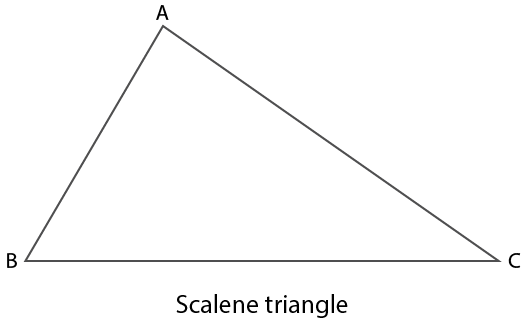(ii) Isosceles triangle has one line of symmetry.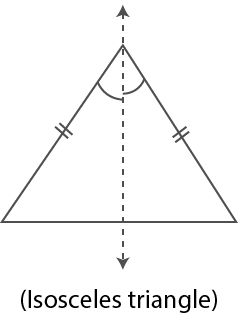(iii) Not possible.

(iv) Equilateral triangle has three lines of symmetry.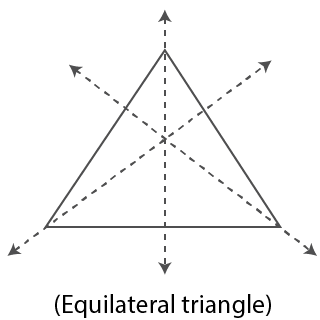(v) Not possible.

5. Draw a quadrilateral with :

(i) no line of symmetry.

(ii) only one line of symmetry.

(iii) exactly two lines of symmetry.

(iv) exactly three lines of symmetry.

(v) exactly four lines of symmetry.

(vi) more than four lines of symmetry.

In each case, if possible, represent the line (s) of symmetry by dotted lines. Also, write the special name of the quadrilateral drawn.

Solution:

(i)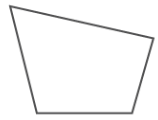(ii)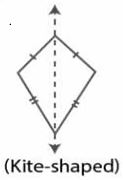(iii)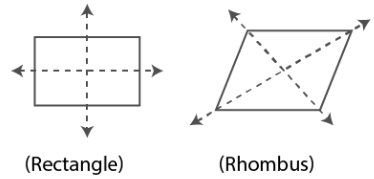(iv) It is not possible.

(v)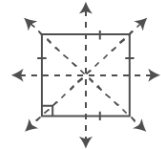(Square)

(vi) It is not possible.

6. Construct an equilateral triangle with each side 6 cm. In the triangle drawn, draw all the possible lines of symmetry.

Solution:

Steps of Construction

1. Construct a line segment BC = 6 cm.

2. Taking B and C as centres and 6 cm radius construct two arcs which intersect each other at the point A.

3. Now join AB and AC.

∆ ABC is the required equilateral triangle.

4. Construct the angle bisectors of ∠A, ∠B and ∠C which are the lines of symmetry.

These are three in number as the triangle is equilateral.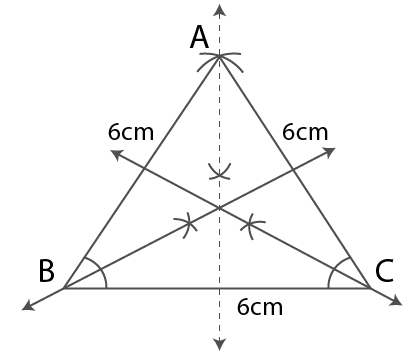7. Construct a triangle ABC in which AB = AC = 5cm and BC = 5.6 cm. If possible, draw its line (s) of symmetry.

Solution:

Steps of Construction

1. Construct a line segment BC = 5.6 cm.

2. Taking B and C as centres and 5 cm radius construct two arcs which intersect each other at the point A.

3. Now join AB and AC.

∆ ABC is the required isosceles triangle.

4. Construct the bisector of ∠A which is the line of symmetry.

There is only one line of symmetry as the triangle is an isosceles triangle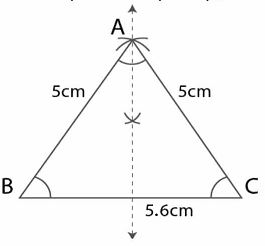8. Construct a triangle PQR such that PQ = QR = 5 .5 cm and angle PQR = 90°. If possible, draw its lines of symmetry.

Solution:

It is given that

angle PQR = 90°

∠P = ∠R

Since the opposite sides are equal we get

∠P + ∠R = 900

Here ∠P = ∠R = 90/2 = 450

Steps of Construction

1. Construct a line segment QR = 5.5 cm.

2. At the point Q construct a ray which makes an angle 900 and cut off QP = 5.5 cm.

3. Now join PR.

∆ PQR is an isosceles triangle.

4. Construct an angle bisector of ∠PQR which is the line of symmetry.

It has only one line of symmetry as the triangle is isosceles.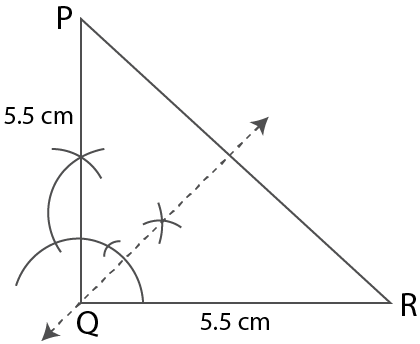9. If possible, draw a rough sketch of a quadrilateral which has exactly two lines of symmetry.

Solution:

It is given that the quadrilateral has exactly two lines of symmetry.

So it must be a rhombus or a rectangle.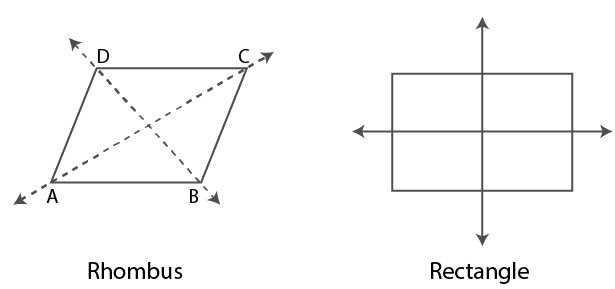10. A quadrilateral ABCD is symmetric about its diagonal AC. Name the sides of this quadrilateral which are equal.

Solution:

It is given that